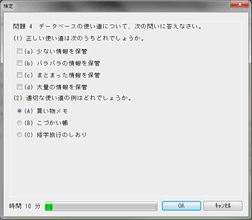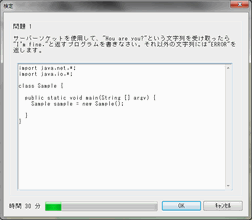\$B"#(B C\$B8@8l%3!<%9!!35MW(B

C\$B8@8l\$O!"\$9\$Y\$F\$N%W%m%0%i%_%s%0\$N4pK\\$G\$9!#(B \$B

\$B\$7\$+\$7!"\$@\$+\$i\$H\$\$\$C\$F8B\$i\$l\$?\$3\$H\$7\$+\$G\$-\$J\$\$\$o\$1\$G\$O\$"\$j\$^\$;\$s!#(B C\$B8@8l\$O2?\$G\$b\$G\$-\$kK|G=8@8l\$H\$\$\$C\$F\$h\$\$\$G\$7\$g\$&!#9bB.\$+\$D%3%s%Q%/%H\$KF0\$/\$?\$a!"AH\$_9~\$_7O\$d%2!<%`%=%U%H\$G\$O\$[\$H\$s\$I\$,(BC\$B8@8l\$r;HMQ\$7\$F\$\$\$^\$9!#(B

\$BEv%3!<%9\$OI8=`#3%v7n\$G=\$N;\$7\$^\$9!#65:`\$O#3:}\$K\$o\$+\$l\$F\$*\$j!"3NG'%F%9%H\$O#32s\$G\$9!#(B \$B:G8e\$KB46H8!Dj\$,\$"\$j\$^\$9\$N\$G!"ET9g#4\$D\$N;n83\$K%Q%9\$7\$J\$1\$l\$P\$J\$j\$^\$;\$s!#(B \$B;n83FbMF\$O%3%s%T%e!<%?\$N4pACCN<1!"(BC\$B\$NJ8K!\$NCN<1!"\$*\$h\$S%W%m%0%i%_%s%0\$G\$9!#(B

\$B"#(B \$B65:`9=@.(B

\$B65:`%?%\$%H%k(B\$B>O(B\$BFbMF(B
12\$B:M\$+\$i\$N(BC\$B8@8l(B
\$BBh#1J,:}(B
\$BBh#1>O!!%3%s%T%e!<%?\$N4pAC(B \$B!&%3%s%T%e!<%?\$NNr;K(B
\$B!&%3%s%T%e!<%?\$N\$7\$/\$_(B
\$B!?J?t\$H#1#6?J?t(B
\$BBh#2>O!!%W%m%0%i%_%s%08@8l(B \$B!&%W%m%0%i%_%s%08@8l(B
\$B!&%3%s%Q%\$%i\$H%\$%s%?!<%W%j%?(B
\$B!&%3%s%Q%\$%k\$N%W%m%;%9(B
\$BBh#3>O!!\$O\$8\$a\$F\$_\$h\$&(B \$B!&%3%s%Q%\$%iF~ \$B!&%;%C%H%"%C%W(B
\$B!&%3%s%Q%\$%k\$N\$7\$+\$?(B
\$BBh#4>O!!2qOC\$9\$k%W%m%0%i%`(B \$B!&%W%m%0%i%`\$N9=B\$(B
\$B!&%3%s%=!<%k\$+\$i\$NF~=PNO(B
\$B!&%G%P%C%0J}K!(B
\$BBh#5>O!!J8(B \$B!&@k8@J8\$H \$B!&JQ?t\$H7?(B
\$B!&%G!<%?\$NFbItI=8=(B
\$BBh#6>O!!4X?t(B

\$B!&I8=`4X?t(B
\$B!&<+:n4X?t(B
\$B!&J,3d%3%s%Q%\$%k(B
\$B!&%3%^%s%I%i%\$%sF~NO(B

12\$B:M\$+\$i\$N(BC\$B8@8l(B
\$BBh#2J,:}(B
\$BBh#7>O!!%W%m%0%i%_%s%0!&%9%?%\$%k(B \$B!&%W%m%0%i%_%s%0!&%9%?%\$%k(B
\$B!&%\$%s%?!<%U%'!<%9(B
\$B!&%0%m!<%P%kJQ?t(B
\$BBh#8>O!!%]%\$%s%?(B \$B!&%]%\$%s%?\$H\$O(B
\$B!&%]%\$%s%?\$NA`:n(B
\$BBh#9>O!!G[Ns(B \$B!&G[Ns\$H\$O(B
\$B!&G[Ns\$H7?(B
\$B!&J8;zNsG[Ns(B
\$B!&G[Ns\$X\$N%"%/%;%9(B
\$BBh#1#0>O!!1i;;;R(B \$B!&Ey9f(B
\$B!&;;=Q1i;;;R(B
\$B!&BeF~1i;;;R(B
\$B!&O@M}1i;;;R(B
\$B!&%S%C%H1i;;;R(B
\$B!&%"%I%l%91i;;;R(B
\$B!&1i;;\$N5,B'(B
\$BBh#1#1>O!!@)8fJ8(B \$B!&(Bfor\$B%k!<%W(B
\$B!&(Bwhile\$B%k!<%W(B
\$B!&>r7oJ,4t(B
12\$B:M\$+\$i\$N(BC\$B8@8l(B
\$BBh#3J,:}(B
\$B!!(B
\$BBh#1#2>O!!%U%!%\$%kF~=PNO(B \$B!&%U%!%\$%k\$N(Bopen\$B\$H(Bclose
\$B!&FI\$_9~\$_\$H=PNO(B
\$B!&%9%H%j!<%`(B
\$B!&%F%-%9%H%b!<%I(B
\$BBh#1#3>O!!9=B\$BN\$H6&MQBN(B \$B!&9=B\$BN(B
\$B!&6&MQBN(B
\$B!&(Btypedef
\$BBh#1#4>O!!%a%b%j\$N3NJ]\$H3+J|(B \$B!&(Bmalloc\$B\$H(Bfree
\$B!&F0E*%a%b%j(B
\$BBh#1#5>O!!%G!<%?9=B\$\$N\$\$\$m\$\$\$m(B \$B!&Fs \$B!&%]%\$%s%?G[Ns(B
\$B!&O"7k%j%9%H(B
\$B!&%9%?%C%/(B
\$B!&%O%C%7%eI=(B
\$B!&%S%C%H%U%#!<%k%I(B

\$B"#(B \$B2hLL%5%s%W%k(B

\$B65:`\$N%5%s%W%k2hLL(B\$B8!Dj\$N%5%s%W%k2hLL(B